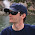## Wednesday, September 13, 2017

### Amount to Words conversion at Acumatica Reports

Hi Everyone,

As we do not yet have Amount to Words function inside our top notch Report Designer, let me share one created to print cheques from AR module.

Same logic applies and can be reused anywhere inside Acumatica Report Designer. I did it for up to 10,000 amount, but you can easily extend it upwards.

1. First of all lets create few Variables on top of the Reporting Group where we are going to use A2W conversion.

a) AmountCents - that will be total amount converted to Cents. I used ARPayment.CuryOrigDocAmt but you can use ANY other numeric field as a SOURCE
b) AmtThousands - that will be just how many thousands we have
c) AmtHundreds - displays number of hundreds only
d) AmtOnes - Ones only
c) AmtCents - Cents only

Below are all the formulae:

AmountCents:
=CInt([ARPayment.CuryOrigDocAmt]*100)

AmtThousands:
=Floor(\$AmountCents/100000)

AmtHundreds:
=Floor((\$AmountCents-\$AmtThousands*100000)/10000)

AmtTens:
=Floor((\$AmountCents-\$AmtThousands*100000-\$AmtHundreds*10000)/1000)

AmtOnes:
=Floor((\$AmountCents-\$AmtThousands*100000-\$AmtHundreds*10000-\$AmtTens*1000)/100)

AmtCents:
=CInt(Right(CStr(\$AmountCents),2))

2. Secondly add quite long formula into the field itself, here it is:

Your Field: =Iif(\$AmtThousands<>0,Switch(\$AmtThousands=10,'Ten',\$AmtThousands=9,'Nine',\$AmtThousands=8,'Eight',\$AmtThousands=7,'Seven',\$AmtThousands=6,'Six',\$AmtThousands=5,'Five',\$AmtThousands=4,'Four',\$AmtThousands=3,'Three',\$AmtThousands=2,'Two',\$AmtThousands=1,'One') + ' Thousand ','') + Iif(\$AmtHundreds<>0,Switch(\$AmtHundreds=9,'Nine',\$AmtHundreds=8,'Eight',\$AmtHundreds=7,'Seven',\$AmtHundreds=6,'Six',\$AmtHundreds=5,'Five',\$AmtHundreds=4,'Four',\$AmtHundreds=3,'Three',\$AmtHundreds=2,'Two',\$AmtHundreds=1,'One') + ' Hundred ','') + Iif(\$AmtTens<>0,Switch(\$AmtTens=9,'Ninety',\$AmtTens=8,'Eighty',\$AmtTens=7,'Seventy',\$AmtTens=6,'Sixty',\$AmtTens=5,'Fifty',\$AmtTens=4,'Forty',\$AmtTens=3,'Thirty',\$AmtTens=2,'Twenty',\$AmtTens=1,'') + ' ','') + Iif(\$AmtTens=1,Switch(\$AmtOnes=9,'Nineteen',\$AmtOnes=8,'Eighteen',\$AmtOnes=7,'Seventeen',\$AmtOnes=6,'Sixteen',\$AmtOnes=5,'Fifteen',\$AmtOnes=4,'Fourteen',\$AmtOnes=3,'Thirteen',\$AmtOnes=2,'Twelve',\$AmtOnes=1,'Eleven',\$AmtOnes=0,'Ten') + ' ','') + Iif(\$AmtTens<>1,Switch(\$AmtOnes=9,'Nine',\$AmtOnes=8,'Eight',\$AmtOnes=7,'Seven',\$AmtOnes=6,'Six',\$AmtOnes=5,'Five',\$AmtOnes=4,'Four',\$AmtOnes=3,'Three',\$AmtOnes=2,'Two',\$AmtOnes=1,'One',\$AmtOnes=0,''),'') + Iif(\$AmountCents=\$AmtCents,'Zero','') + Iif(\$AmtCents=0,' Only',' and ' + CStr(\$AmtCents) + '¢ Only')

3. Let see what we got:

It works only up to 10k, but if you wish, you can add in front of the formula further parsing.

All the Best,
Sergey.

1.Hi Sergey,

I am trying to do amount to words conversion in Acumatica report the way you mentioned above and it works up to 10k. But I want to add the amount as 999,999,999.00 USD in report & so I extended the number but it is not worked yet. That's why,Is it possible to add on AR report? Or Can you please share the formula for this amount if possible?
Thank you very much in advance

Regards,
Julie

1.Hi Julie,
Please share your formula I will check.

2.Good day,
In order to make this in spanish language, what we need to do?

Regards.